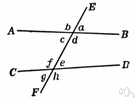# interior angle

Also found in: Thesaurus, Encyclopedia, Wikipedia.
Related to interior angle: exterior angle
interior angle
Angles 3, 4, 5, and 6 are interior angles. Angles 3 and 6 are alternate interior angles, as are angles 4 and 5.

## interior angle

n.
1. Any of the four angles formed between two straight lines intersected by a third straight line.
2. An angle formed inside a polygon by two adjacent sides.
American Heritage® Dictionary of the English Language, Fifth Edition. Copyright © 2016 by Houghton Mifflin Harcourt Publishing Company. Published by Houghton Mifflin Harcourt Publishing Company. All rights reserved.

## interior angle

n
1. (Mathematics) an angle of a polygon contained between two adjacent sides
2. (Mathematics) any of the four angles made by a transversal that lie inside the region between the two intersected lines
Collins English Dictionary – Complete and Unabridged, 12th Edition 2014 © HarperCollins Publishers 1991, 1994, 1998, 2000, 2003, 2006, 2007, 2009, 2011, 2014

## inte′rior an′gle

n.
1. an angle formed between parallel lines by a third line that intersects them.
2. an angle formed within a polygon by two adjacent sides.
Random House Kernerman Webster's College Dictionary, © 2010 K Dictionaries Ltd. Copyright 2005, 1997, 1991 by Random House, Inc. All rights reserved.

## in·te·ri·or angle

(ĭn-tîr′ē-ər)
1. Any of the four angles formed inside two straight lines when these lines are intersected by a third straight line.
2. An angle formed by two adjacent sides of a polygon and included within the polygon. Compare exterior angle.
The American Heritage® Student Science Dictionary, Second Edition. Copyright © 2014 by Houghton Mifflin Harcourt Publishing Company. Published by Houghton Mifflin Harcourt Publishing Company. All rights reserved.
ThesaurusAntonymsRelated WordsSynonymsLegend:
 Noun 1interior angle - the angle inside two adjacent sides of a polygoninternal angleangle - the space between two lines or planes that intersect; the inclination of one line to another; measured in degrees or radiansreentering angle, reentrant angle - an interior angle of a polygon that is greater than 180 degrees
Based on WordNet 3.0, Farlex clipart collection. © 2003-2012 Princeton University, Farlex Inc.
References in periodicals archive ?
Mark 10 inches on each side and bend an interior angle of approximately 110 degrees.
Programming Challenge 1 required students to use their knowledge of geometry content by focusing on the properties of squares--including the number of sides and interior angle measures.
Another question also tackled geometry by asking students to compute the interior angle inside a circle superimposed over Kim's butt.
Thanks to the gravity-defying lean of the building, each room in the tower is different, as is each pane of faE*ade glass and every interior angle.
Initially, they defined it as a figure made with two half lines, but they were not satisfied with this definition because it was not clear whether the definition referred to interior angle or exterior angle (see Figure 2).
Then, notice that [[angle].sub.P]AOB is equal to the interior angle [angle]P of the spherical triangle [delta]APB, because the tangent plane of [S.sup.2] at P is orthogonal to the line OP.
deflection angle interior angle chord 3-4-5 method tape-sine method sine cosine tangent clockwise counterclockwise azimuth bearing INTRODUCTION
Students determine the interior angle measurement in degrees for six polygons, without using a protractor but with access to Pentablocks (see fig.
The Turn machine then turns the turtle (by the supplement of the polygon's interior angle).
If we simply draw the location of F on the flat pentagon before expansion and look at the triangle ABF, the interior angle at F will be 360 [degrees]/5 = 72 [degrees], while that at A or B is 324 [degrees]/6 = 54 [degrees].
The traverse is completed by moving the instrument to consecutive corners, zero-setting the instrument on the previous corner and turning the interior angle. Setting up the instrument on each corner makes it easy to measure distance between corners by stadia or EDM.
The angle with any other vertex which lies on this line (for example, angle A[C.sub.1]B) will be less than angle ACB because [angle]A[C.sub.1]B < [angle]A[A.sub.1]B (the exterior angle A[A.sub.1]B of the triangle B[A.sub.1][C.sub.1] is bigger than the remote interior angle [angle]A[C.sub.1]B) and [angle]A[A.sub.1]B = [angle]ACB (inscribed angles).

Site: Follow: Share:
Open / Close